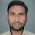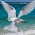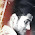# GATE Questions - OS - Synchronization

Previous GATE questions with solutions on Operating Systems (Synchronization) - CS/IT

GATE-1996
1. A critical section is a program segment
(a) which should run in a certain specified amount of time
(c) where shared resources are accessed
(d) which must be enclosed by a pair of semaphore operations, P and V

Ans: option (c)

GATE-1996
2. A solution to the Dining Philosophers Problem which avoids deadlock is
(a) ensure that all philosophers pick up the left fork before the right fork
(b) ensure that all philosophers pick up the right fork before the left fork
(c) ensure that one particular philosopher picks up the left fork before the right fork, and that all other philosophers pick up the right fork before the left fork
(d) None of the above

Ans: option (c)

GATE-2010
3. Consider the methods used by processes P1 and P2 for accessing their critical sections whenever needed, as given below. The initial values of shared boolean variables S1 and S2 are randomly assigned.
 Method Used by P1 Method Used by P2 while (S1 == S2) ; Critica1 Section S1 = S2; while (S1 != S2) ; Critica1 Section S2 = not (S1);

Which one of the following statements describes the properties achieved?
(a) Mutual exclusion but not progress
(b) Progress but not mutual exclusion
(c) Neither mutual exclusion nor progress
(d) Both mutual exclusion and progress

Ans: option (a)
Explanation:
Principle of Mutual Exclusion: No two processes may be simultaneously present in the critical section at the same time. That is, if one process is present in the critical section other should not be allowed.
P1 can enter critical section only if S1 is not equal to S2, and P2 can enter critical section only if S1 is equal to S2. Therefore Mutual Exclusion is satisfied.

Progress: no process running outside the critical section should block the other interested process from entering critical section whenever critical section is free.
Suppose P1 after executing critical section again want to execute the critical section and P2 dont want to enter the critical section, then in that case P1 has to unnecesarily wait for P2. Hence progress is not satisfied.

GATE-1998
4. A counting semaphore was initialized to 10. Then 6 P (wait) operations and 4V (signal) operations were completed on this semaphore. The resulting value of the semaphore is
(a) 0    (b) 8     (c) 10     (d) 12

Ans: option(b)
Explanation:
6P => decrements the semaphore 6 times. Hence , the value becomes 4.
4V => increments the semaphore 4 times. Hence , the value becomes 8.
Note: The positive value of counting semaphore indicates that those many down (P) operations can be carried out successfully. The negative value of counting semaphore indicates that the number of blocked processes.

GATE-2000
5. Let m…m be mutexes (binary semaphores) and P …. P be processes.
Suppose each process P[i] executes the following:
wait (m[i]);wait (m[(i+1) mode 4]);
.........
release (m[i]); release (m[(i+1)mod 4]);
This could cause
(c) Starvation, but not deadlock     (d) None of the above

Ans: option (b)
Explanation:
Deadlock occurs, if each each process gets preempted after executing wait(m[i]);

GATE-2009
6. The enter_CS() and leave_CS() functions to implement critical section of a process are realized using test-and-set instruction as follows:
void enter_CS(X)
{
while test-and-set(X) ;
}
void leave_CS(X)
{
X = 0;
}
In the above solution, X is a memory location associated with the CS and is initialized to 0. Now consider the following statements:
I. The above solution to CS problem is deadlock-free
II. The solution is starvation free.
III. The processes enter CS in FIFO order.
IV More than one process can enter CS at the same time.
Which of the above statements is TRUE?
(a) I only
(b) I and II
(c) II and III
(d) IV only

Ans: option (a)
Explanation:
The test-and-set instruction is an instruction used to write to a memory location and return its old value as a single atomic (i.e., non-interruptible) operation. Since it is an atomic instruction it guarantees mutual exclusion.
Reference: http://en.wikipedia.org/wiki/Test-and-set

GATE-2010
7. The following program consists of 3 concurrent processes and 3 binary semaphores. The semaphores are initialized as S0=1, S1=0, S2=0.
 Process P0 Process P1 Process P2 while (true) { wait (S0); print (0); release (S1); release (S2); } wait (S1); Release (S0); wait (S2); release (S0);

How many times will process P0 print '0'?
(a) At least twice                              (b) Exactly twice
(c) Exactly thrice                              (d) Exactly once

Ans: option (a)
Explanation:
P0 will execute first because only S0=1. Hence it will print 0 (for the first time). Also P0 releases S1 and S2. Since S1=1 and S2=1, therefore P1 or P2, any one of them can be executed.
Let us assume that P1 executes and releases S0 (Now value of S0 = 1). Note that P1 process is completed.
Now S0=1 and S2=1, hence either P0 can execute or P2 can execute. Let us check both the conditions:-

1. Let us assume that P2 executes, and releases S0 and completes its execution. Now P0 executes; S0=0 and prints 0 (i.e. second 0). And then releases S1 and S2. But note that P1 and P2 processes has already finished their execution. Again if P0 tries to execute it goes into sleep condition because S0=0. Therefore, minimum number of times '0' gets printed is 2.

2. Now, let us assume that P0 executes. Hence S0=0, (due to wait(S0)), and it will print 0 (second 0) and releases S1 and S2. Now only P2 can execute, because P1 has already completed its execution and P0 cannot execute because S0 = 0. Now P2 executes and releases S0 (i.e. S0=1) and finishes its execution. Now P0 starts its execution and again prints 0 (thrid 0) and releases S1 and S2 (Note that now S0=0). P1 and P2 has already completed its execution therefore again P1 takes its turn, but since S0=0, it goes into sleep condition. And the processes P1 and P2 which could wakeup P0 has already finished their execution.Therefore, maximum number of times '0' gets printed is 2.

GATE-2012
8. Fetch_And_Add(X,i) is an atomic Read-Modify-Write instruction that reads the value of memory location X, increments it by the value i, and returns the old value of X. It is used in the pseudocode shown below to implement a busy-wait lock. L is an unsigned integer shared variable initialized to 0. The value of 0 corresponds to lock being available, while any non-zero value corresponds to the lock being not available.
AcquireLock(L){
L = 1;
}
ReleaseLock(L){
L = 0;
}
This implementation
(a) fails as L can overflow
(b) fails as L can take on a non-zero value when the lock is actually available
(c) works correctly but may starve some processes
(d) works correctly without starvation

Ans: option (b)
Explanation:
Assume that L=0, that means the lock is now available. A process P1 wants to acquire the lock by executing the AcquireLock() function. We can see that the while loop fails because the Fetch_And_Add instruction returns the previous value of L, which was 0. (Note that after the execution of the atomic instruction, the present value of L is now 1). Since the returned value was 0, the while loop fails and P1 process comes out of the while loop and hence acquires the lock.
Now scheduler schedules another process P2 and context switching takes place. P2 tries to acquire the lock by executing the AcquireLock() function. But it goes on executing the while loop infinitely (because the atomic instruction always returns a non-zero value and the while loop condition is always true).
After some time, scheduler again schedules P1. We assume that P2 was stopped soon after the Fetch_And_Add() instruction returned the value. Hence L has some non-zero value.  Now P1 releases the lock L. That means now L=0. Assume that again context switch takes place and P2 arrives.
We have assumed that P2 was switched out when it executed the Fetch_And_Add instruction and a non-zero value has been returned. Since the returned value was non-zero  the condition becomes true and the statement L=1 is executed. Hence now L=1. Again the while loop is executed, Fetch_And_Add instruction will return value 1 and hence again the while loop enters into infinite loop. Now none of the process is able to acquire the lock. Therefore the above implementation fails.

GATE-2007
9. Two processes, P1 and P2, need to access a critical section of code. Consider the following synchronization construct used by the processes:
 /* P1 */ while (true) {   wants1 = true;   while (wants2 == true);   /* Critical     Section */   wants1=false; } /* Remainder section */ /* P2 */ while (true) {   wants2 = true;   while (wants1==true);   /* Critical     Section */   wants2 = false; } /* Remainder section */
Here, wants1 and wants2 are shared variables, which are initialized to false. Which one of the following statements is TRUE about the above construct?
(a) It does not ensure mutual exclusion.
(b) It does not ensure bounded waiting.
(c) It requires that processes enter the critical section in strict alternation.
(d) It does not prevent deadlocks, but ensures mutual exclusion.

Ans: option (d)
Explanation:
The code ensures the condition of mutual exclusion: Assume P1 is initiated. It sets wants1=true.  Now since wants2 = false, P1 exists from its while loop and enters its critical section. Now suppose context switch takes place and P2 gets executed. Now it sets wants2=true, and now enters the while loop and remains busy till P1 comes out of the critical section and sets wants1=false, because wants1=true( as set by P1). So we can see that the mutual exclusion condition is satisfied.

The code does not prevent deadlock: Assume that P1 starts its execution. It sets wants1=true and then gets preempted. Now P2 starts its execution. P2 sets wants2=true and suddenly gets preempted. Now P1 starts execution; it enters the while loop and finds that wants2=true and remains busy in the while loop. Now P1 gets preempted. P2 enters into execution; it enters the while loop and finds that wants1=true remains busy in the while loop. Hence both P1 and P2 remains busy forever.

GATE-2006
10. The atomic fetch-and-set x, y instruction unconditionally sets the memory location x to 1 and fetches the old value of x in y without allowing any intervening access to the memory location x. consider the following implementation of P and V functions on a binary semaphore .
void P (binary_semaphore *s) {
unsigned y;
unsigned *x = &(s->value);
do {
fetch-and-set x, y;
} while (y);
}
void V (binary_semaphore *s) {
S->value = 0;
}
Which one of the following is true?
(a) The implementation may not work if context switching is disabled in P.
(b) Instead of using fetch-and-set, a pair of normal load/store can be used
(c) The implementation of V is wrong
(d) The code does not implement a binary semaphore

Ans: option (a)
Explanation:
Assume that P1 process enters the P function. Initially if the value of semaphore is 1, according to the definition of the fetch-and-set instruction, the value of y will be 1  when the fetch-and-set instruction is executed. Hence the while loop goes on executing until other processes execute the V function. But if context switching is disabled in P while loop will keep on executing and other processes are not allowed to execute.

GATE-2006
Barrier is a synchronization construct where a set of processes synchronizes globally i.e. each process in the set arrives at the barrier and waits for all others to arrive and then all processes leave the barrier. Let the number of processes in the set be three and S be a binary semaphore with the usual P and V functions. Consider the following C implementation of a barrier with line numbers shown on left.
void barrier (void) {
1: P(S);
2: process_arrived++;
3: V(S);
4: while (process_arrived !=3);
5: P(S);
6: process_left++;
7: if (process_left==3) {
8: process_arrived = 0;
9: process_left = 0;
10: }
11: V(S);
}
The variables process_arrived and process_left are shared among all processes and are initialized to zero. In a concurrent program all the three processes call the barrier function when they need to synchronize globally.

11. The above implementation of barrier is incorrect. Which one of the following is true?
(a) The barrier implementation is wrong due to the use of binary semaphore S
(b) The barrier implementation may lead to a deadlock if two barrier in invocations are used in immediate succession.
(c) Lines 6 to 10 need not be inside a critical section
(d) The barrier implementation is correct if there are only two processes instead of three.

Ans: option (b)
Explanation:
It is mentioned that there are 3 processes. Let it be P1, P2 & P3.  Every process arrives at the barrier, that is it completes its first section of the code. Hence process_arrived = 3. Now assume that P1 continues its execution and leaves the barrier and makes process_left=1. Now assume that P1 again invokes the barrier function immediately. At this stage, process_arrived =4. P1 now waits. Soon P2 leaves the barrier, which makes process_left=2. Now P3 leaves the barrier which makes process_left=3. "If" condition is true and now process_arrived and process_left is reset to 0.
We know that P1 is waiting. At this stage assume that P2 invokes the barrier function, hence process_arrived=1 and it waits. P3 invokes the barrier function, hence process_arrived=2. All process have reached the barrier but since the process_arrived=2, all processes keeps on waiting. Hence deadlock.

12. Which one of the following rectifies the problem in the implementation?
(a) Lines 6 to 10 are simply replaced by process_arrived--
(b) At the beginning of the barrier the first process to enter the barrier waits until process_arrived becomes zero before proceeding to execute P(S).
(c) Context switch is disabled at the beginning of the barrier and re-enabled at  the end.

Ans: option (b)

1.thanku it wwas very useful :-)

2.thank you........ :)

3.I have a doubt with question 8.. Answer should be D.
L is a shared variable. So when P1 release the lock, L=0. This means when the while condition in P2 is executed again , Fetch&add(x,1) will return 0. Condition fails, process comes out of while loop and acquires lock.

4.much needed explanation..heartiest regards

5.Nice information shared of GATE question. Look some more GATE previous papers according to topic wise and year wise.

6.7.8.thank u it was vry usefull

9.in fetch _and_add que option 1 should also be right .. make me clear please

10.question no .6 will contain (b) as answer.Lets suppose there is a process p1 which is executing enter_cs() ans set x=1 now a process P2 comes this will go into infinite while loop in between if P1 has completed leave_cs() also and another process p3 came and saw x=0 and started executing the enter_cs() whereas P2 is still is in infinite loop,so this may cause of starvation that p2 is not getting chance.

1.The solution is not starvation free as you said. We have to select TRUE statement , so II is false. Option(a) is the right answer

11.question no 8 --b option is correct as proved .. one proof is sufficient to make the entire statement false which has already been proved there.. that it would fail ... so there is no chance of it being successful

12.grate job keep it up...need people like U

13.Hello Sir,
I am confuse between MSc Maths after IIT-JAM or do M.tech in Mathematics after GATE.
Can you tell me the pros and cons for both the exam. I am so puzzled.

14.Beautiful Collection..

15.NICE...COLLECTION....
VERY USEFULL

1.16.This comment has been removed by the author.

17.liked very much this btechonline.org

18.https://www.geeksforgeeks.org/gate-gate-cs-2015-set-3-question-20/
for the same type example they said it prevents deadlock and fail to ensure mutual exclusion!!